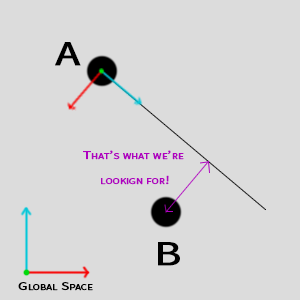# "How far to the local left/right is another object"?

The title pretty much sums it up. I need to inplement a bit of code which will check the distance from object A to object B, but only on one axis: the local x axis of object A.I guess the answer is simple, yet I’m a bit confused.

You need to project a vector of (B - A) onto A’s X-axis from the direction of (presumably) A’s Z-axis as the normal.

Eno Khan’s comment, after a few tweaks, works fine!

Vector projection is based on the idea that a vector (i.e. B - A) is projected onto another vector (A’s local X-axis). The Z-axis, being perpendicular to the X-axis, would inherently be the part removed by this projection.

For instance:

``````// (B - A)
Vector3 distBetween = (B.transform.position - A.transform.position);
// A's local X-Axis
Vector3 xAxis = A.transform.right;
// Vector projection on A's Z-Axis (assuming the Y-Axis isn't a factor
Vector3 xAxisDist = Vector3.Project(distBetween, xAxis);
``````

If the Y-axis is factored in and is not desired, you could instead set the Y value in “distBetween” to 0.